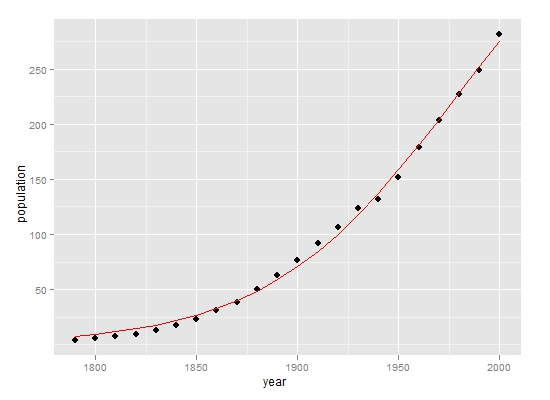# 用nls函数进行非线性回归

1 9,389

## 一、模型拟合

```library(car)
pop.mod1 <- nls(population ~ theta1/(1+exp(-(theta2+theta3*year))),start=list(theta1 = 400, theta2 = -49, theta3 = 0.025), data=USPop, trace=T)
summary(pop.mod)```

`pop.mod2 <- nls(population ~ SSlogis(year,phi1,phi2,phi3),data=USPop)`

## 二、判断拟合效果

```library(ggplot2)
p <- ggplot(USPop,aes(year, population))
p+geom_point(size=3)+geom_line(aes(year,fitted(pop.mod1)),col='red')```<

## 三、残差诊断

`plot(fitted(pop.mod1) , resid(pop.mod1),type='b')`

• 本文由 发表
• 转载请务必保留本文链接：https://www.plob.org/article/3612.html

• 大米儿5217 0

您好，除AIC之外还有R2可以用于比较模型的拟合效果吗？在R中应该怎么计算R2呢？